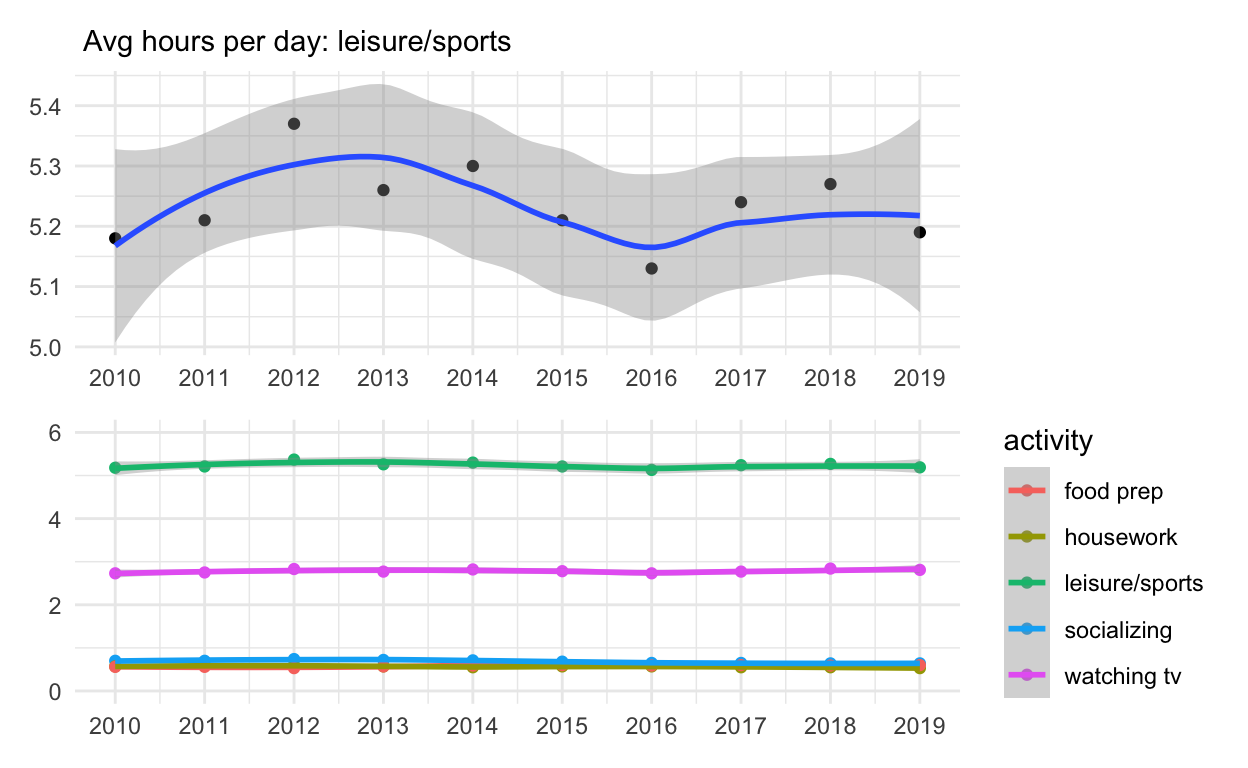# Exploratory-analysis-II

A short description of the post.

1. Load the R package we will use.

library(tidyverse) library(patchwork)

``````library(tidyverse)
``````
1. Quiz questions
• Replace all the ???s. These are answers on your moodle quiz. Run all the individual code chunks to make sure the answers in this file correspond with your quiz answers

• After you check all your code chunks run then you can knit it. It won’t knit until the ??? are replaced

• The quiz assumes that you have watched the videos, downloaded (to your examples folder) and worked through the exercises in exercises_slides-50-61.Rmd

1. Pick one of your plots to save as your preview plot. Use the ggsave command at the end of the chunk of the plot that you want to preview.

# Question: modify slide 51

• Create a plot with the mpg dataset
• assign the variable displ to the x-axis
• assign the variable hwy to the y-axis
• add facet_wrap to split the data into panels based on the manufacturer
``````ggplot(mpg) +
geom_point(aes(x = displ, y = hwy)) +
facet_wrap(vars(class))
``````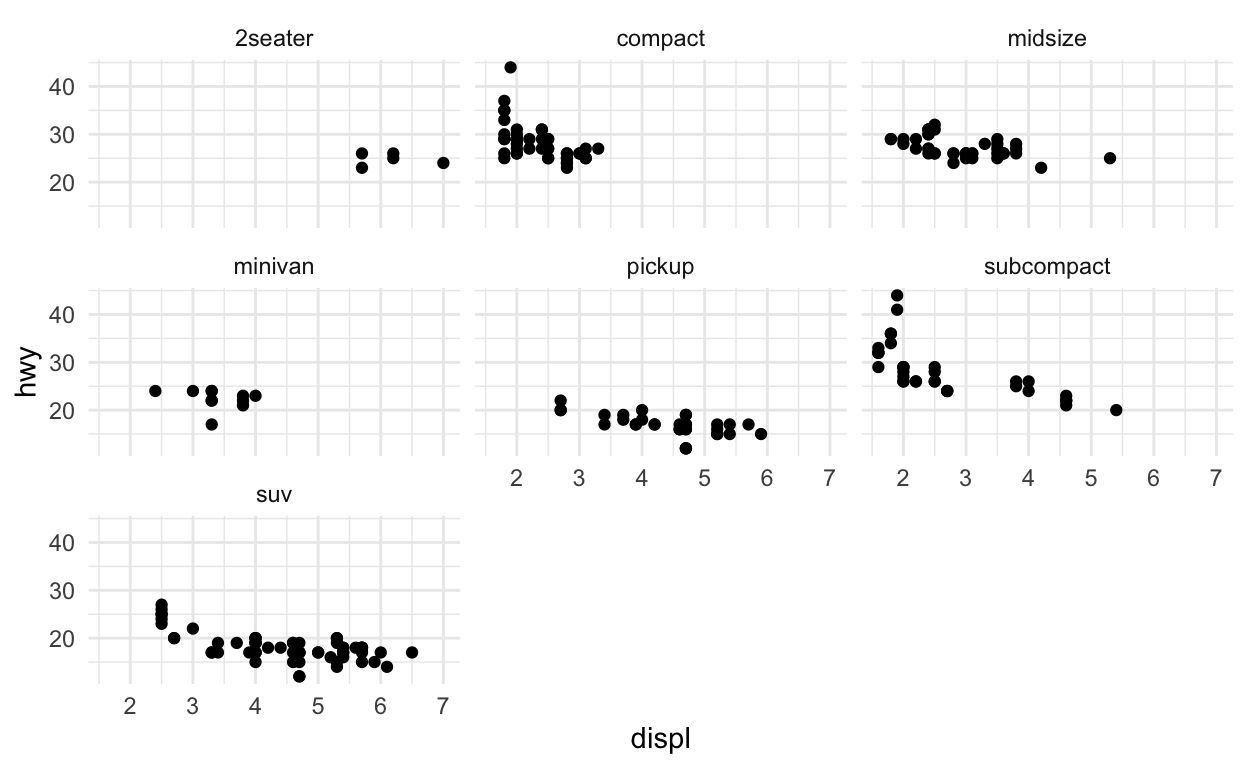# Question: modify facet-ex-2

• Create a plot with the mpg dataset
• add bars with with geom_bar
• assign the variable manufacturer to the y-axis
• add facet_grid to split the data into panels based on the class
• let scales vary across columns
• let space taken up by panels vary by columns
``````## See https://ggplot2.tidyverse.org/reference/facet_grid.html

ggplot(mpg) +
geom_bar(aes(y = manufacturer)) +
facet_grid(vars(class), scales = 'free_y', space = 'free_y')
``````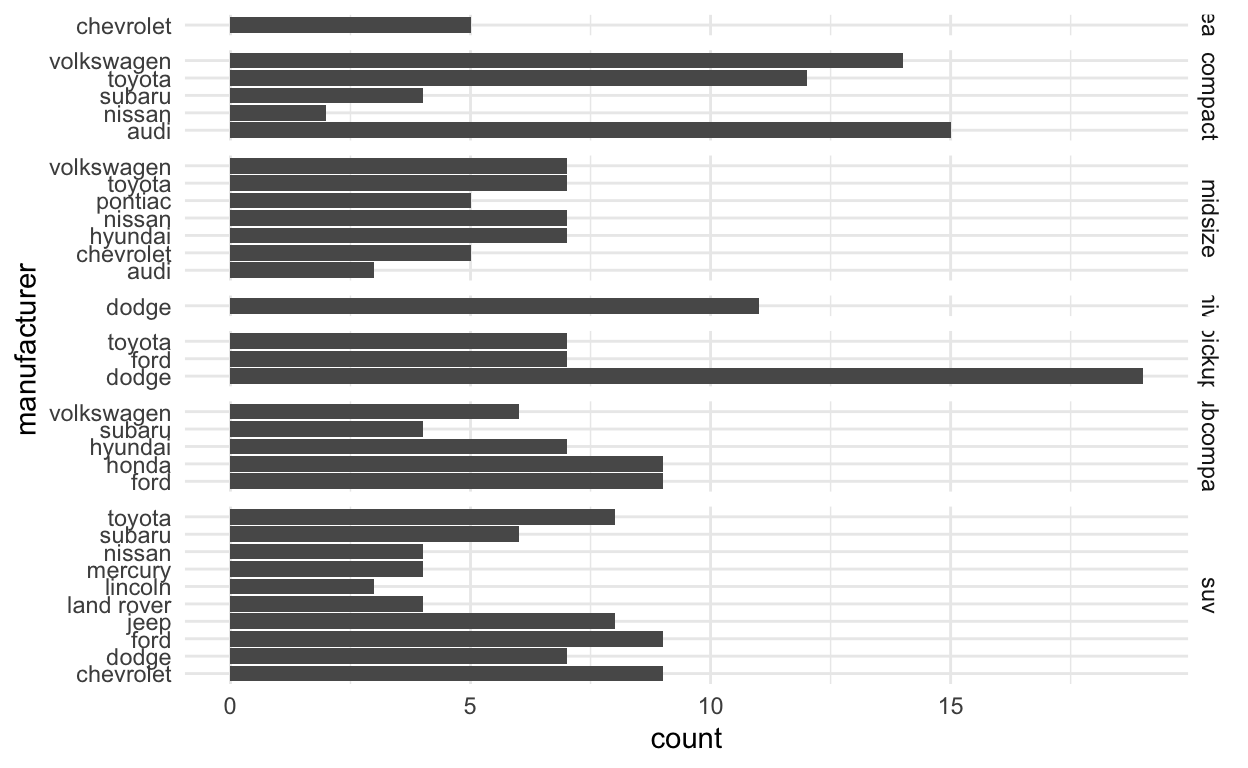# Question: spend_time

the patchwork slides and the vignette: https://patchwork.data-imaginist.com/articles/patchwork.html Download the file spend_time.csv from moodle into directory for this post. Or read it in directly:

spend_time contains 10 years of data on how many hours Americans spend each day on 5 activities

``````spend_time <- read_csv("https://estanny.com/static/week8/spend_time.csv")
``````

extract observations for SEE QUIZ THEN create a plot with that data ADD a barchart with with geom_col assign activity to the x-axis assign avg_hours to the y-axis assign activity to fill ADD scale_y_continuous with breaks every hour from 0 to 6 hours ADD labs to set subtitle to SEE QUIZ set x and y to NULL so they won’t be labeled assign the output to p1 display p1

``````p1 <- spend_time %>% filter(year == "2018")  %>%
ggplot() +
geom_col(aes(x = activity, y = avg_hours, fill = activity)) +
scale_y_continuous(breaks = seq(0, 6, by = 1)) +
labs(subtitle = "Avg hours per day: 2018", x = NULL, y = NULL)
p1
``````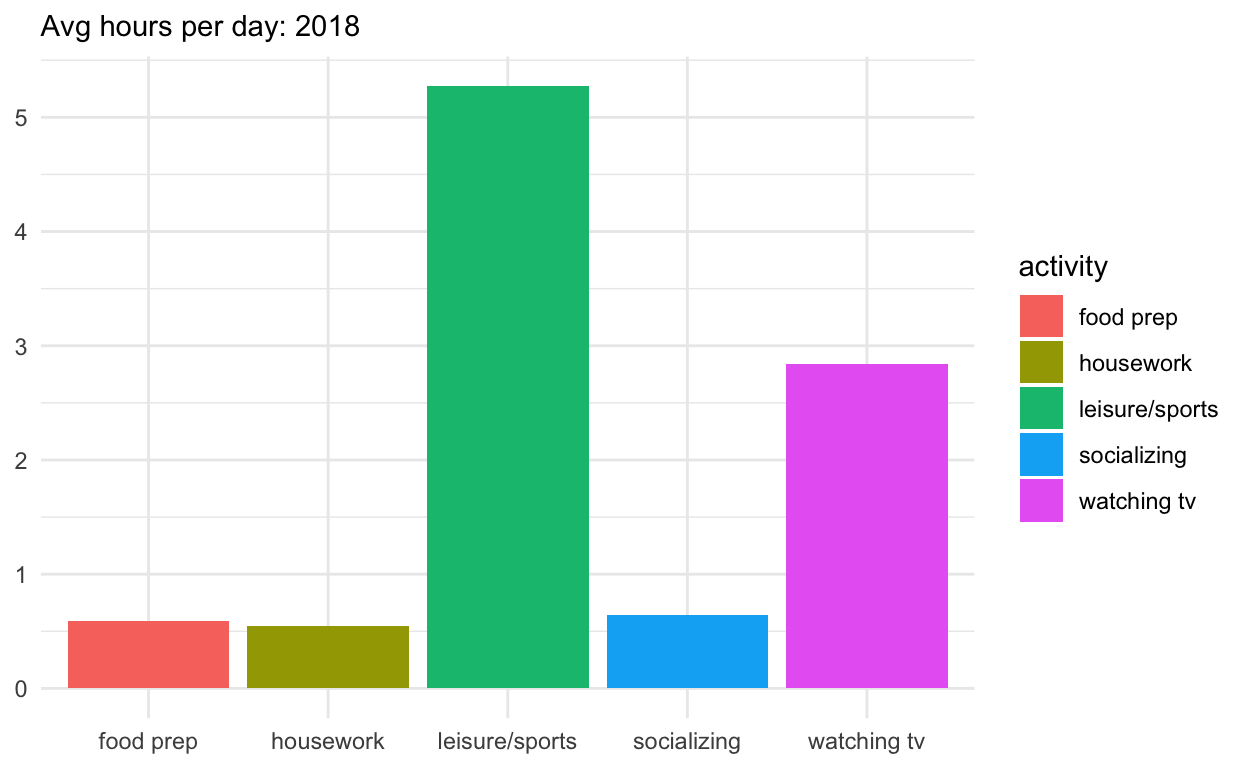THEN create a plot with it ADD a barchart with with geom_col assign year to the x-axis assign avg_hours to the y-axis assign activity to fill ADD labs to set subtitle to “Avg hours per day: 2010-2019” set x and y to NULL so they won’t be labeled assign the output to p2 display p2

``````p2 <- spend_time  %>%
ggplot() +
geom_col(aes(x = year, y = avg_hours, fill = activity)) +
labs(subtitle  = "Avg hours per day: 2010-2019", x = NULL, y = NULL)
p2
``````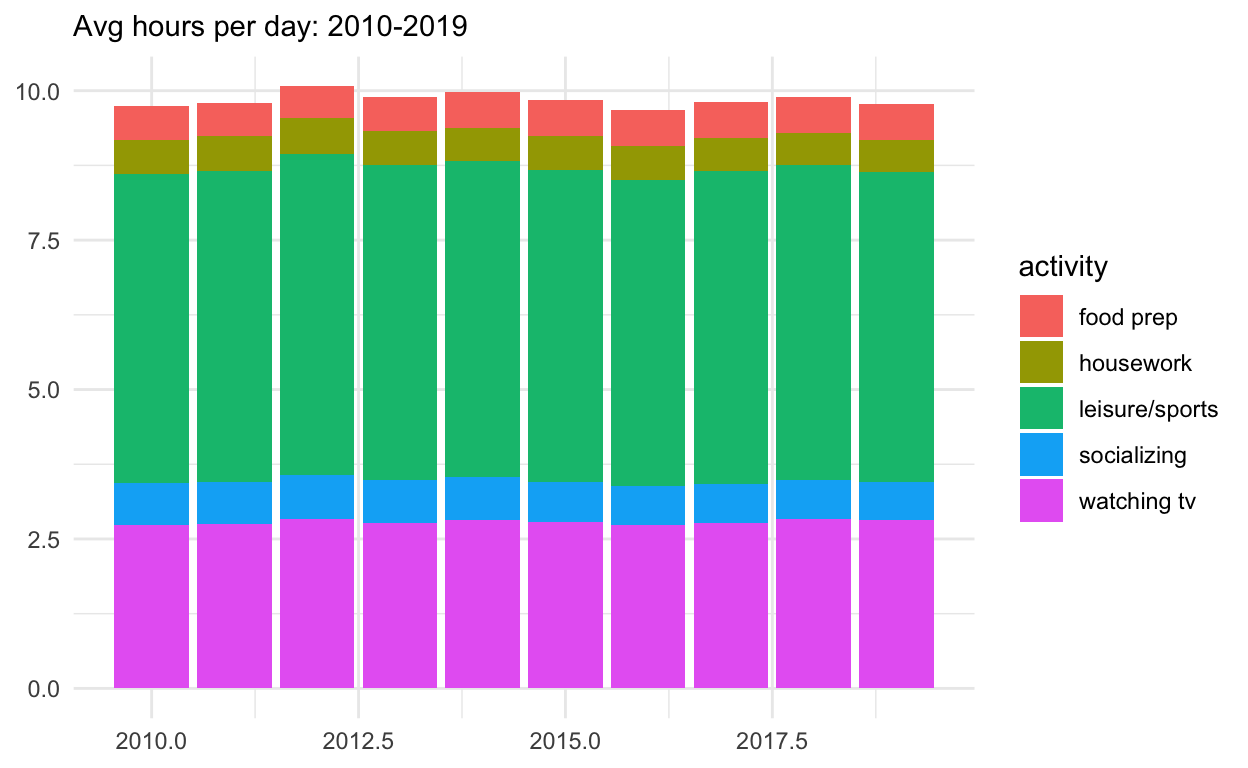Use patchwork to display p1 on top of p2

assign the output to p_all display p_all

``````p_all  <-  p1 / p2
p_all
``````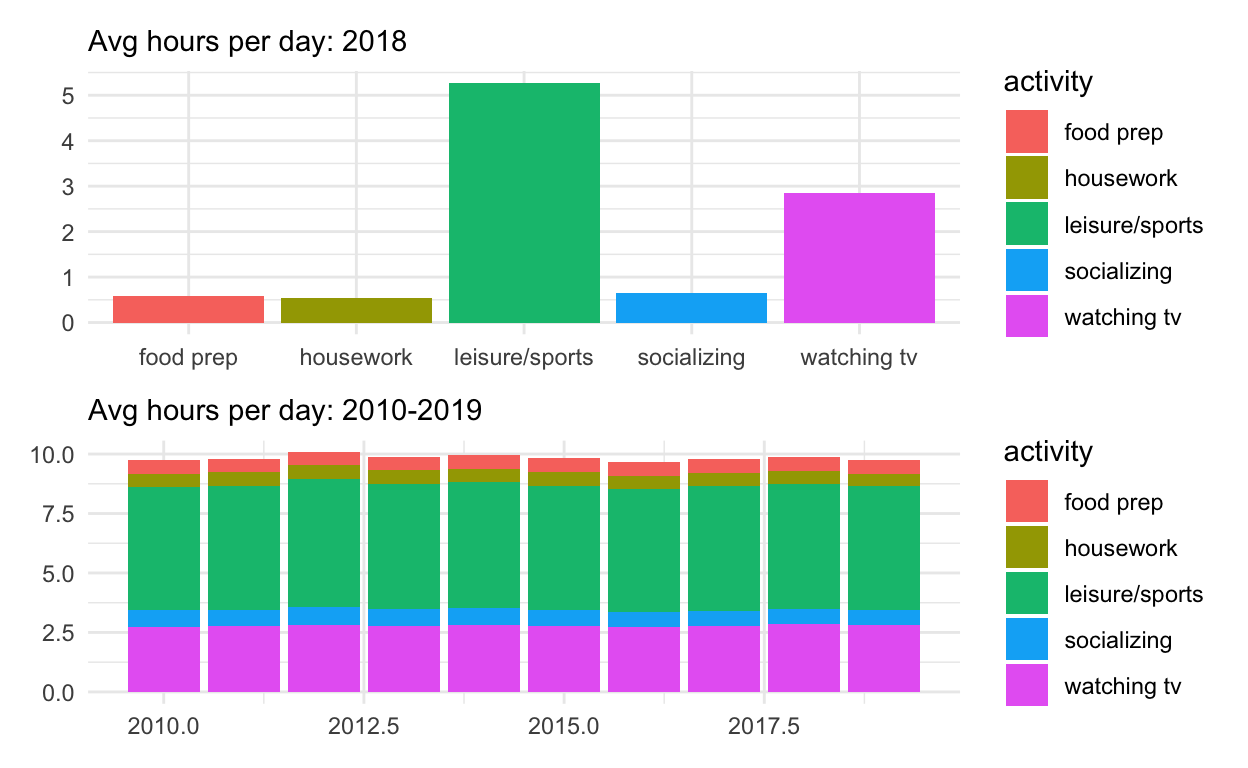AND set legend.position to ‘none’ to get rid of the legend assign the output to p_all_no_legend display p_all_no_legend

``````p_all_no_legend  <- p_all & theme(legend.position = 'none')
p_all_no_legend
``````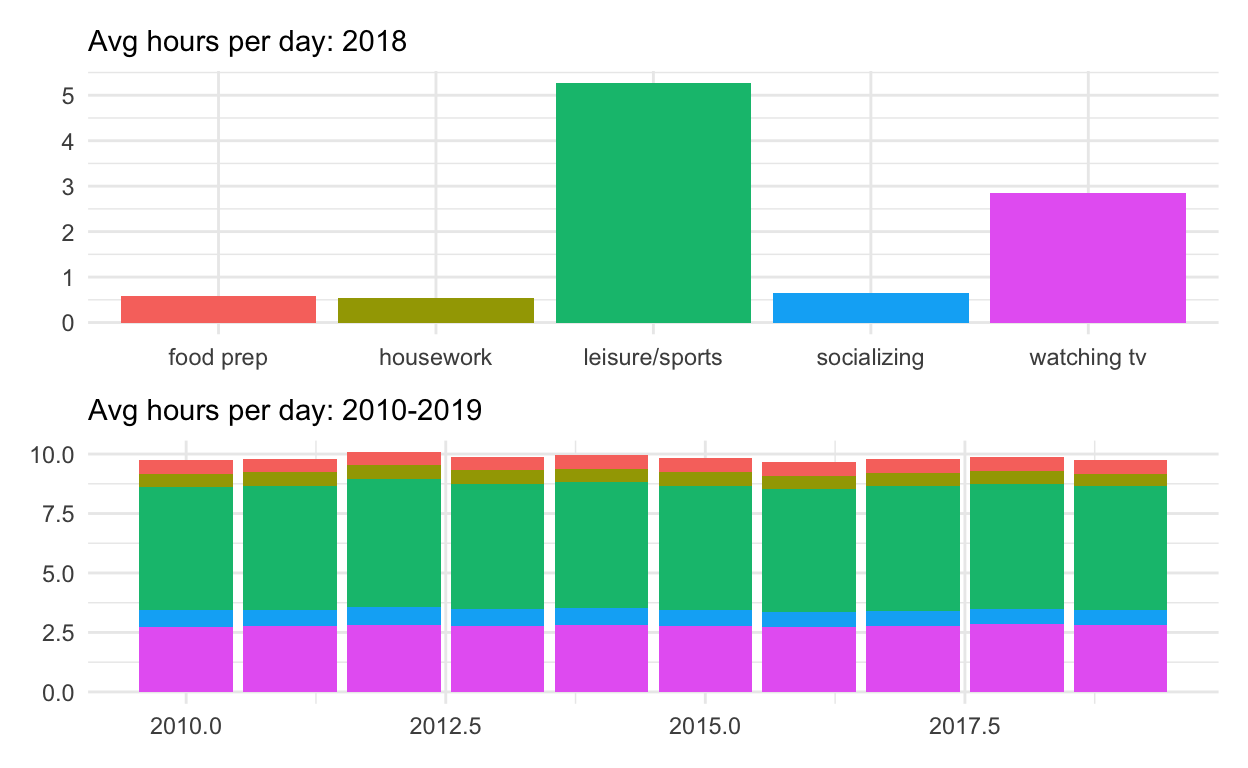see how annotate the composition here: https://patchwork.data-imaginist.com/reference/plot_annotation.html ADD plot_annotation set title to “How much time Americans spent on selected activities” caption to “Source: American Time of Use Survey, https://data.bls.gov/cgi-bin/surveymost?tu

``````p_all_no_legend  +
plot_annotation(title = "How much time Americans spent on selected activities",
caption = "Source: American Time of Use Survey, https://data.bls.gov/cgi-bin/surveymost?tu")
``````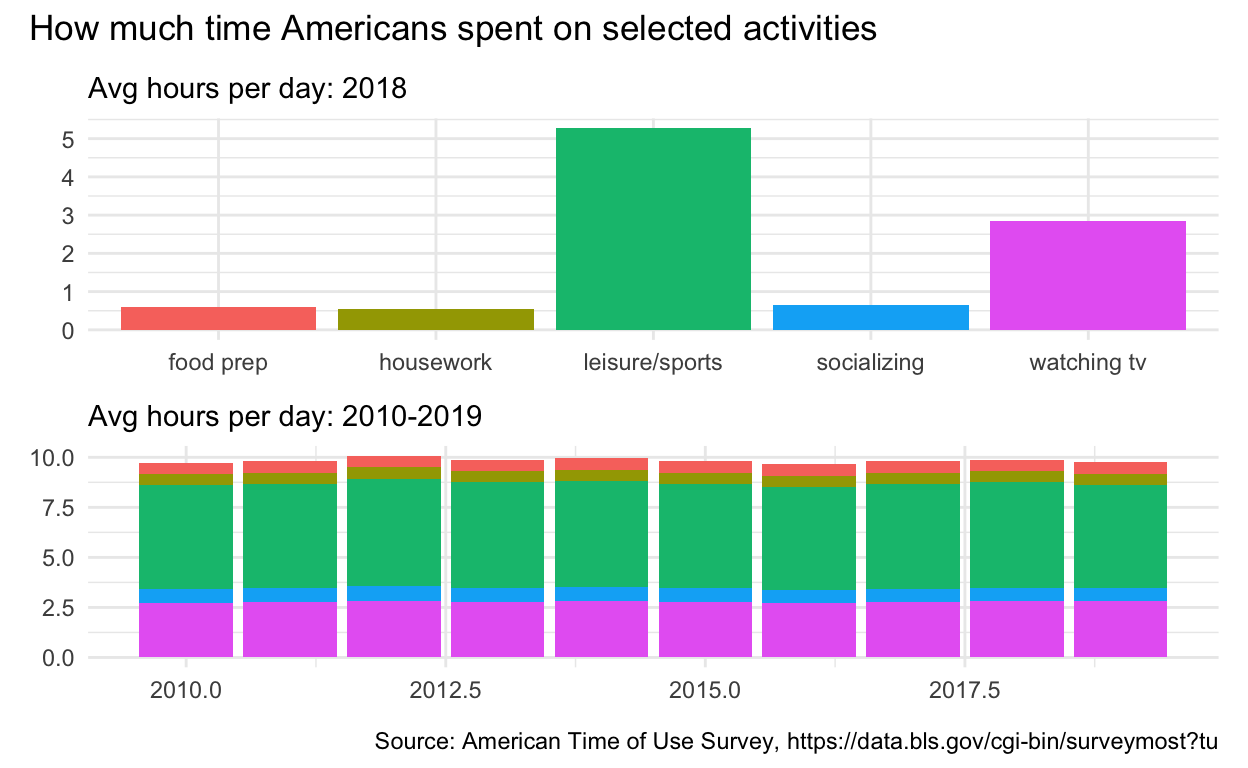# Question: Patchwork 2

use spend_time from last question patchwork slides

extract observations for SEE QUIZ THEN create a plot with that data ADD points with geom_point assign year to the x-axis assign avg_hours to the y-axis ADD line with geom_smooth assign year to the x-axis assign avg_hours to the y-axis ADD breaks on for every year on x axis with with scale_x_continuous ADD labs to set subtitle to SEE QUIZ set x and y to NULL so x and y axes won’t be labeled assign the output to p4 display p4

``````p4  <-
spend_time %>% filter(activity == "leisure/sports")  %>%
ggplot() +
geom_point(aes(x = year, y = avg_hours))  +
geom_smooth(aes( x = year,  y = avg_hours)) +
scale_x_continuous(breaks = seq(2010, 2019, by = 1)) +
labs(subtitle = " Avg hours per day: leisure/sports", x = NULL, y = NULL)

p4
``````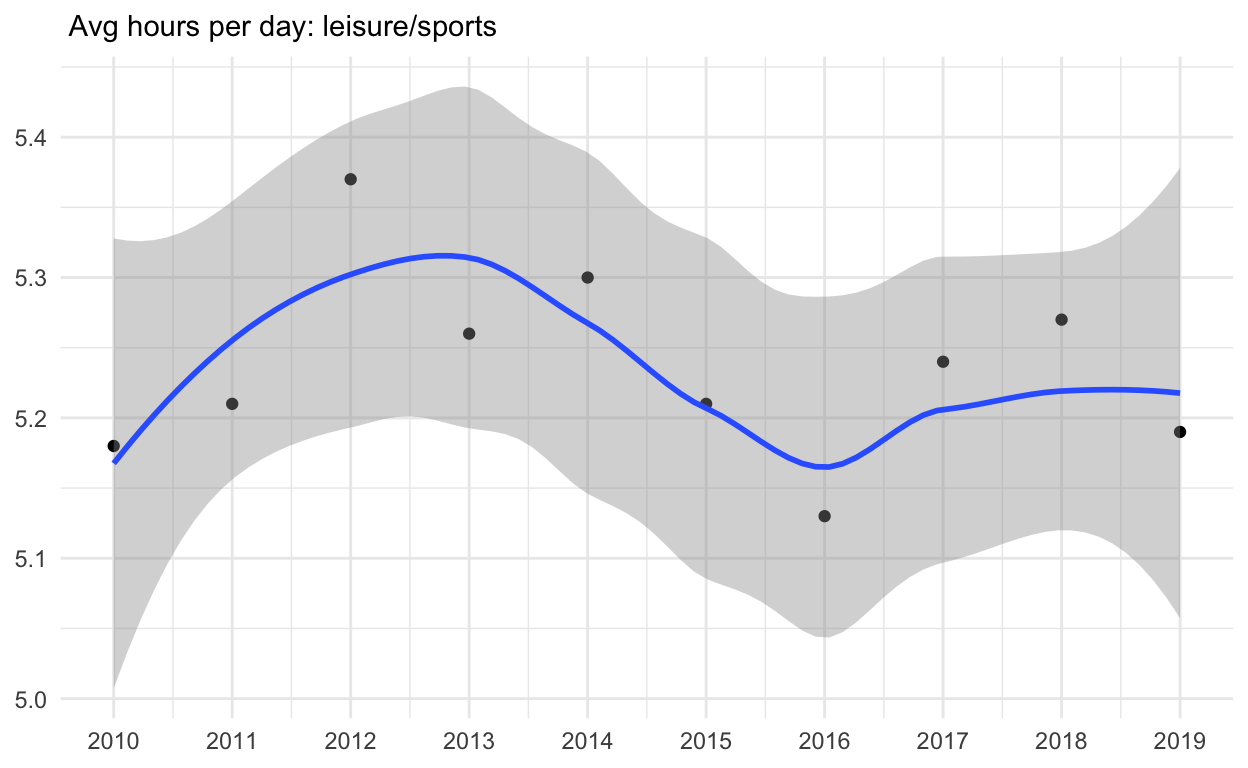ADD coord_cartesian to change range on y axis to 0 to 6 assign the output to p5 display p5

``````p5 <-
p4 + coord_cartesian(ylim = c(0, 6))
p5
``````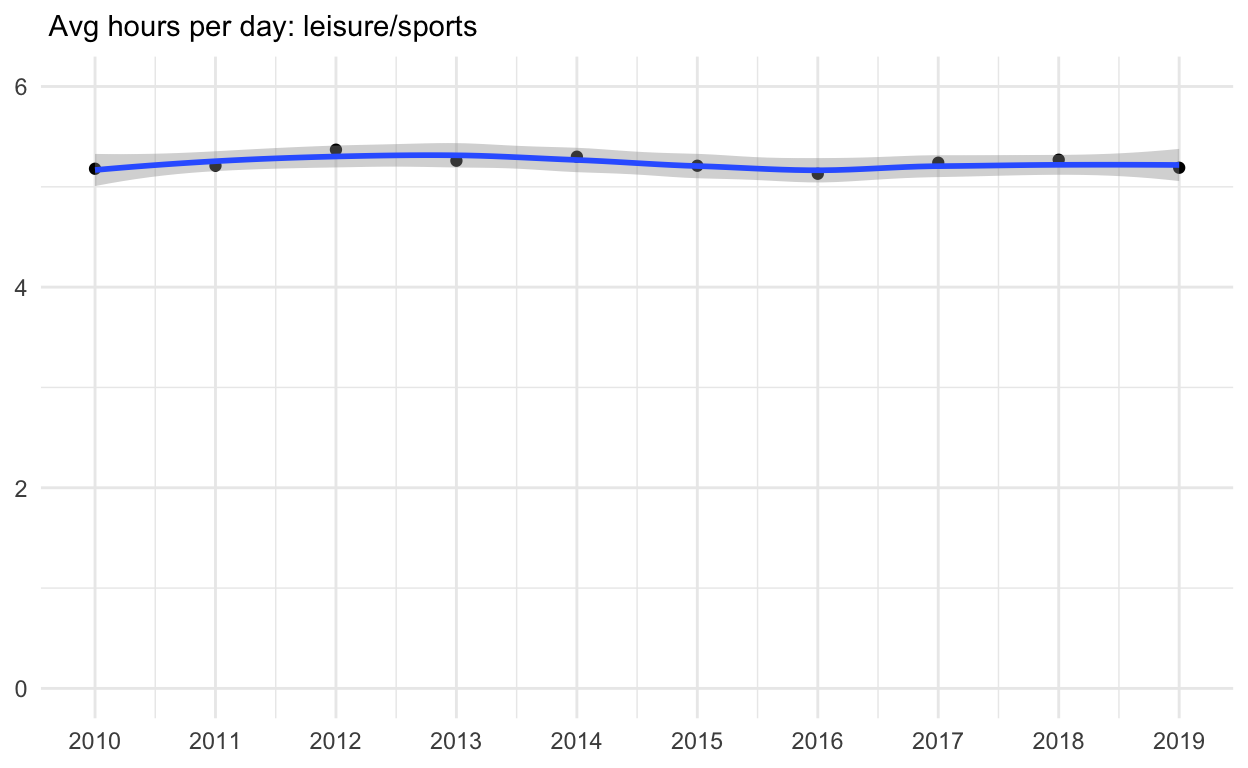create a plot with that data ADD points with geom_point assign year to the x-axis assign avg_hours to the y-axis assign activity to color assign activity to group ADD line with geom_smooth assign year to the x-axis assign avg_hours to the y-axis assign activity to color assign activity to group ADD breaks on for every year on x axis with with scale_x_continuous ADD coord_cartesian to change range on y axis to 0 to 6 ADD labs to set x and y to NULL so they won’t be labeled assign the output to p6 display p6

``````p6   <-
spend_time  %>%
ggplot() +
geom_point(aes(x = year, y = avg_hours, color = activity, group = activity)) +
geom_smooth(aes(x = year, y = avg_hours, color = activity, group = activity)) +
scale_x_continuous(breaks = seq(2010, 2019, by = 1)) +
coord_cartesian(ylim = c(0, 6)) +
labs(x = NULL, y = NULL)

p6
``````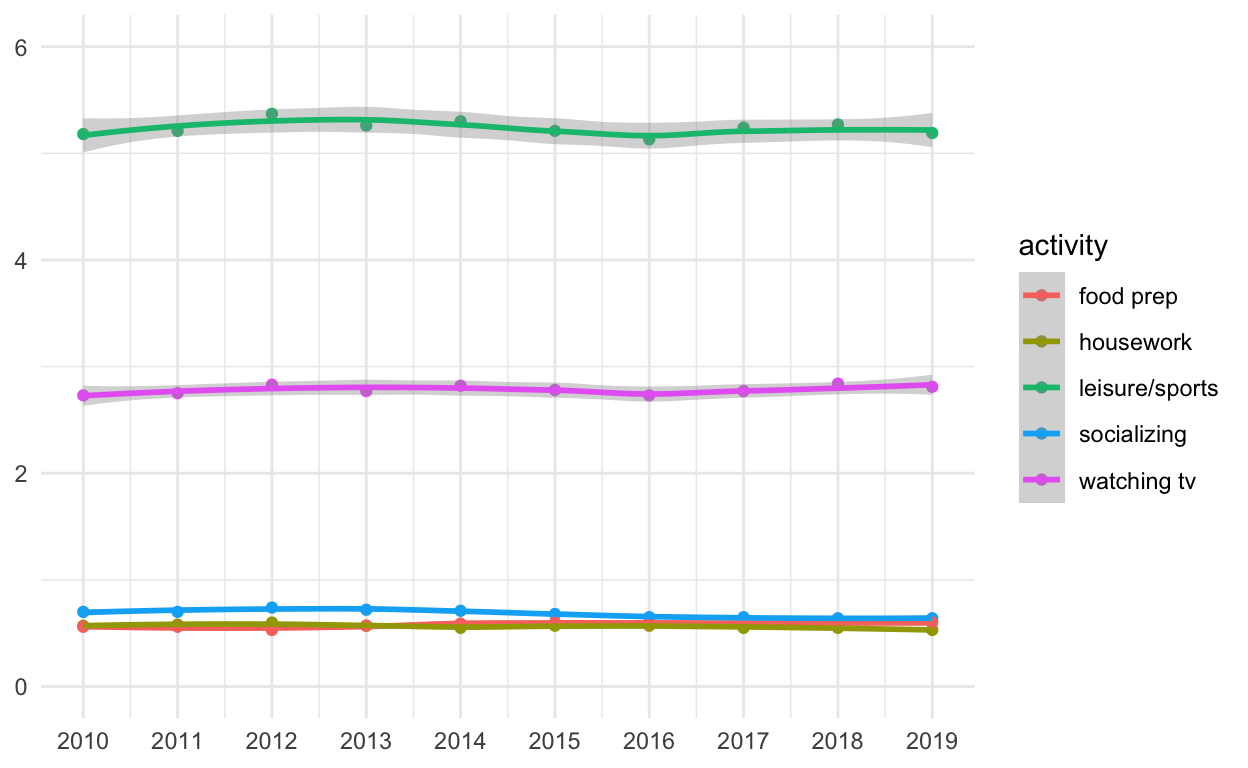Use patchwork to display p4 and p5 on top of p6

``````p_all <- p4 | p5
p4 / p6
``````